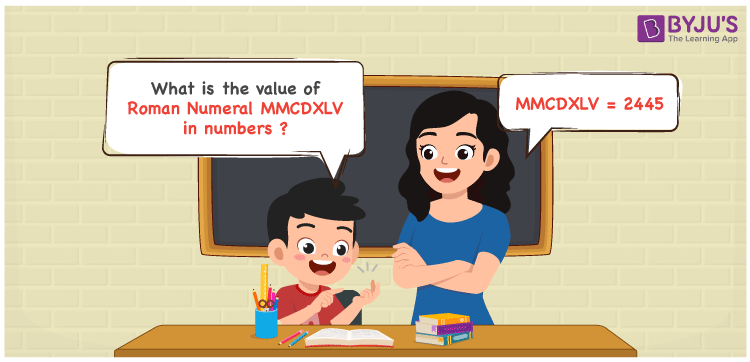Checkout JEE MAINS 2022 Question Paper Analysis : Checkout JEE MAINS 2022 Question Paper Analysis :

MMCDXLV Roman Numerals is 2445. In this article, the roman numerals are split and indicated in numbers in an easy to learn manner. The fundamental concepts in Maths like addition operation have to be focused on by the students. The Roman Numerals conversion is discussed here in an accurate way to enhance problem solving abilities of students. Therefore, MMCDXLV can be indicated as 2445 in numbers.

 Number Roman Numeral 2445 MMCDXLV## How to Write MMCDXLV Roman Numerals in Numbers?

MMCDXLV is converted to numbers by splitting the roman numerals according to the place value of each digit.

MMCDXLV = M + M + (D – C) + (L – X) + V

MMCDXLV = 1000 + 1000 + (500 – 100) + (50 – 10) + 5

MMCDXLV = 2445

## Video Lesson on Roman Numerals## Frequently Asked Questions on MMCDXLV Roman Numerals

### How is MMCDXLV written as 2445 in numbers?

We know that

MMCDXLV = M + M + (D – C) + (L – X) + V

MMCDXLV = 1000 + 1000 + (500 – 100) + (50 – 10) + 5

MMCDXLV = 2445

### Calculate the value of 2500 – 55.

We know that

2500 – 55 = 2445

Hence, the value of 2500 – 55 is 2445 which is written as MMCDXLV.

### Find the remainder we obtain when MMCDXLV is divided by IV.

We know that

MMCDXLV = 2445

IV = 4

So the remainder we obtain when MMCDXLV is divided by IV is I.